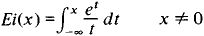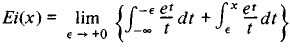Exponential Integral

(redirected from Expint)

exponential integral

[‚ek·spə′nen·chəl ′int·ə·grəl]
(mathematics)
The function defined to be the integral from x to ∞ of (e -t / t) dt for x positive.

Exponential Integral

a special function defined by the integralThis integral cannot be expressed in closed form through elementary functions. If x > 0, then the integral is understood in the sense of its principal value:Site: Follow: Share:
Open / Close